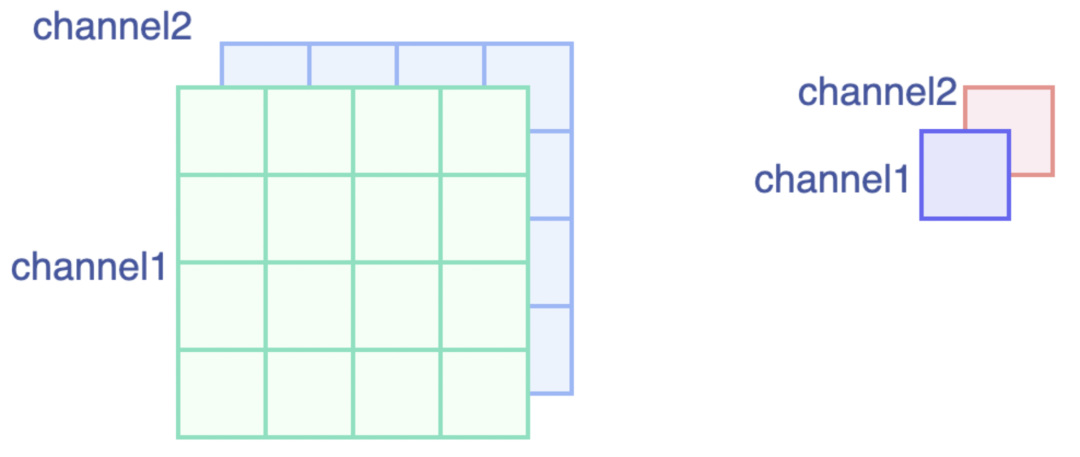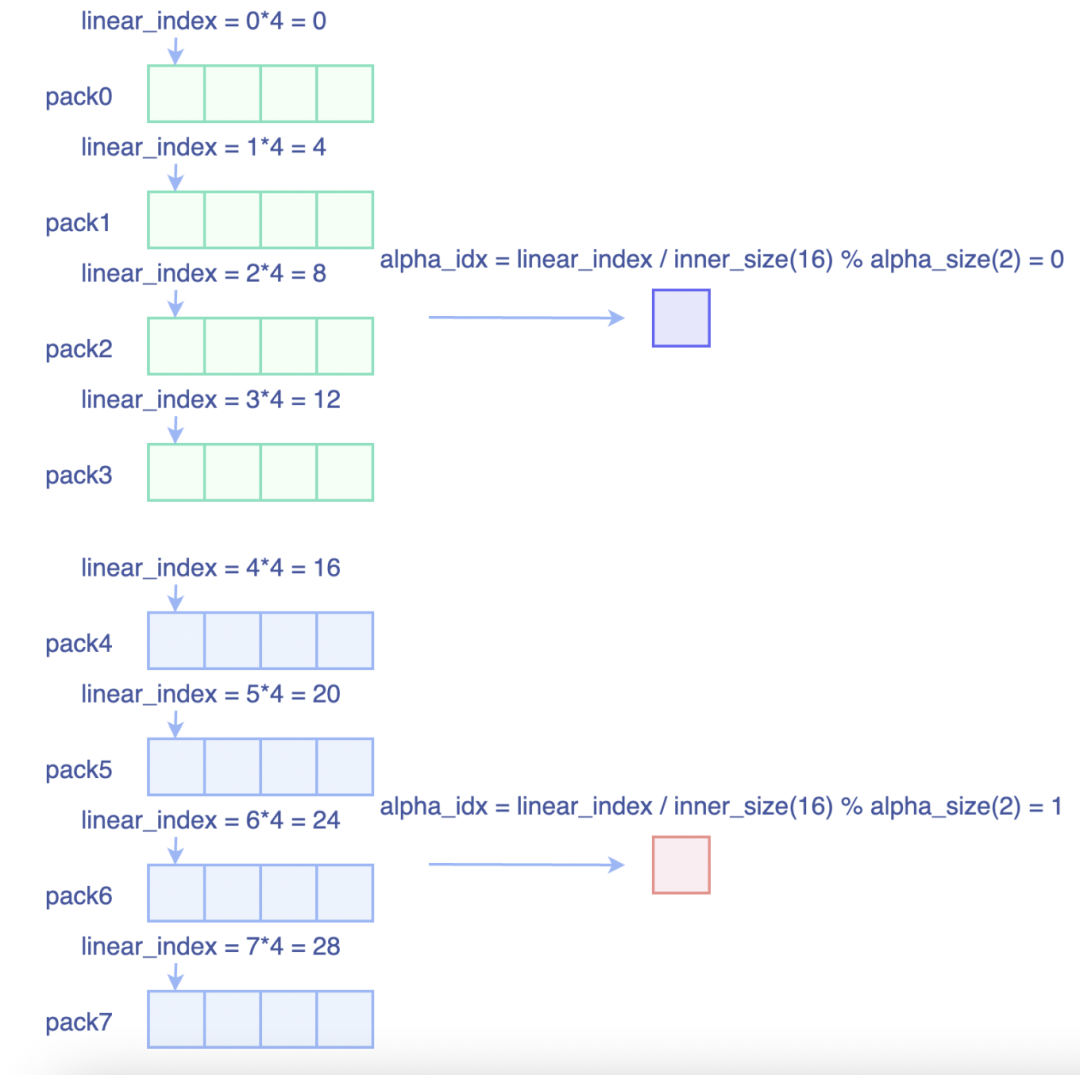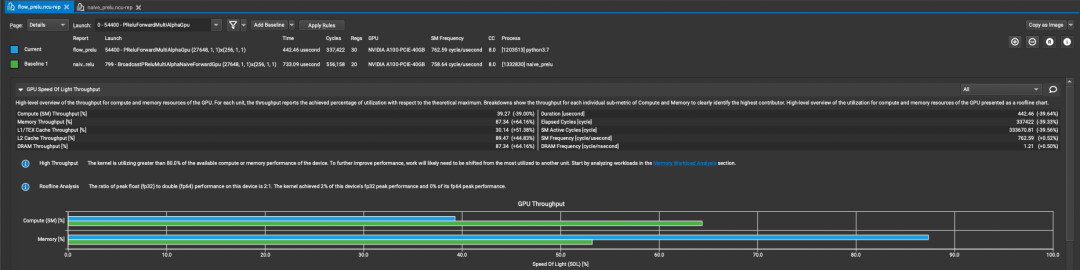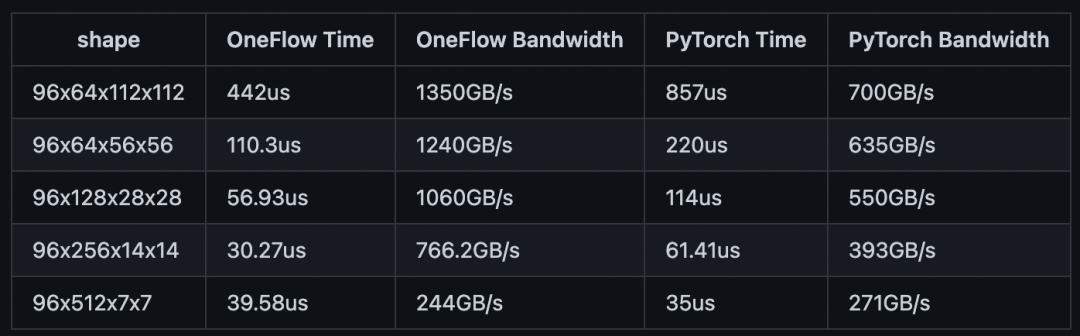# CUDA优化之PReLU性能调优InsightFace模型里大量使用了PReLU激活函数，而PReLU的工作模式有两种：

1. PReLU(1)，此时权重alpha的形状为(1, )，等价于一个Elementwise操作。

2. PReLU(channels)，此时权重alpha的形状为(channels, )，和输入特征(N, C, H, W)中C的大小是对应的。此时PReLU等价于一个Binary Broadcast操作。

1

``template<typename T>``__global__ void PReluForwardGpu(const int32_t elem_cnt, const int32_t alpha_size,``                                const int32_t inner_size, const T* x, const T* alpha, T* y) {``  CUDA_1D_KERNEL_LOOP(i, elem_cnt) {``    const T x_i = x[i];``    const T alpha_i = alpha[(i / inner_size) % alpha_size];``    y[i] = x_i > 0 ? x_i : x_i * alpha_i;``  }``}``

• inner_size表示的是通道维后面维度乘积，以NCHW格式为例，inner_size=H*W

•  alpha_size表示通道维大小

Integer division and modulo operation are costly as they compile to up to 20 instructions.

2

Pack向量化优化````template<typename T, typename IndexType, int pack_size>``__global__ void PReluForwardMultiAlphaGpu(const IndexType elem_cnt, const IndexType alpha_size,``                                          const IndexType inner_size, const T* x, const T* alpha, T* y) {``  int32_t global_thread_id = blockIdx.x * blockDim.x + threadIdx.x;`
`  using LoadType = cuda::elementwise::PackType<T, pack_size>;``  using LoadPack = cuda::elementwise::Pack<T, pack_size>;``  T zero_val = static_cast<T>(0);``  for (int64_t linear_index = global_thread_id * pack_size; linear_index < elem_cnt;``       linear_index += gridDim.x * blockDim.x * pack_size) {``    // 计算当前Pack所使用到Alpha的索引``    ``    IndexType alpha_idx = (linear_index/inner_size%alpha_size);``    const LoadType* x_load = reinterpret_cast<const LoadType*>(x + linear_index);``    // 以向量化的形式加载输入x``    LoadPack x_vec;``    x_vec.storage = *x_load;`
`    LoadPack y_vec;``    // 循环展开，逐个处理Pack内的元素``#pragma unroll``    for (int i = 0; i < pack_size; i++) {``      y_vec.elem[i] = x_vec.elem[i] > zero_val ? x_vec.elem[i] : x_vec.elem[i] * alpha[alpha_idx];``    }``    // 以向量化的形式存储输出y``    *(reinterpret_cast<LoadType*>(y + linear_index)) = y_vec.storage;``  }``}````3